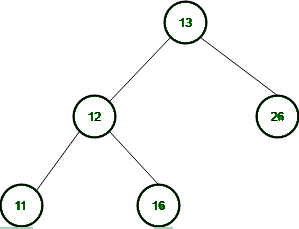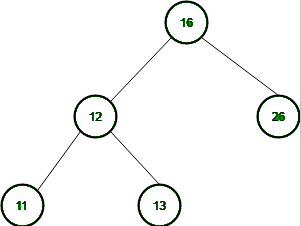# UGC NET COMPUTER SCIENCE SOLVED PAPERS 2014-16 - UGC NET Computer Science Paper 2 JULY 2016

>>>>>>>>UGC NET Computer Science Paper 2 JULY 2016

• A

11• B

12• C

13• D

16• Option : D
• Explanation :
When we delete root node, tree will bebut this tree is not balance, so we will find inorder predecessor or inorder successor and replace it with root node now. In this problem we will use inorder predecessor-Root node will be 16.So, option (D) is correct.

• A

Underflow occurs• B

Stack operations are performed smoothly• C

Overflow occurs• D

None of the above• Option : B
• Explanation :All Stack operations are performed smoothly. So, option (B) is correct.

• A

(n/2)-1• B

n/2 +1• C

(n-1)/2• D

(n + 1)/2• A

Implementation of recursion• B

Evaluation of a postfix expression• C

Job scheduling• D

Reverse a string• Option : C
• Explanation :
We can use stack for string reversal, evaluation of postfix expression and most important is implementation of recursion but job scheduling is not done by stack. So, option (C) is correct.

• A

15• B

14• C

13• D

12